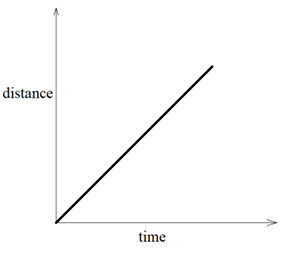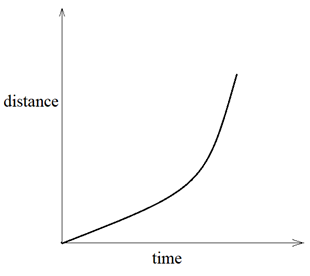# Types of Motion

When a body is moving, it is said to be in motion and when it does not move, it said to be at rest. A body can be moving in many different ways therefore, we have different types of motion. Motion can be classified on various factors namely, dimension, direction, uniformity etc.

## Types of Motion – Video Explanation

### The various types of motion have their own specific characteristics.

#### 1. Translational motion –

The type of motion in which an object moves in single direction at a given time is called translational motion. It is further divided into two parts – rectilinear motion and curvilinear motion.

Rectilinear motion

It is the type of motion in which an object performing translational motion moves on a straight path. For example – cars moving on a straight road, birds flying in a straight path etc.

Curvilinear motion

It is the motion in which an object performing translational motion moves on a curved path. For example – kids cycling on a circular path, car taking turn on highways etc.

#### 2. Rotational motion –

It is the type of motion in which a body moves about an axis. The different parts of the body also move but they cover different distances at a given time. This type of motion can be seen in blades of windmill, merry-go-round in a park etc. When blades of a windmill rotate, the outer portion of its blades move much more distance at a given time but the parts closer to the axis of windmill cover lesser distance.

Rotational motion is also observed in planets. All the planets perform rotational motion about their own axis.

### Motion can also be classified on the basis of its repetition after a particular time period.

#### 3. Periodic motion –

It is the type of motion which repeats itself after a certain period of time. This type of motion is observed in pendulum. The to and fro motion of pendulum repeats every second or we can say that its time period is 1 second. The motion of earth rotating about its own axis is also periodic with time period of 24 hours.

#### 4. Non-periodic motion –

The motion that does not repeat itself at all is called non-periodic motion.  The examples of non-periodic motion are movement of car on a road, bird flying in the sky, children playing and running around in a park.

### Motion – On the basis of dimensions in which an Object travels.

#### 1. One dimensional motion –

It is the type of motion in which the object changes its position with respect to time in only one dimension. This type of motion is observed in a car driving on a road which does not have a turn. The car can only move forwards and backwards on a straight road without taking any turns.

#### 2. Two-dimensional motion –

It is the type of motion in which the object changes its position with respect to time in two dimensions. This type of motion can be seen in the movement of striker in carrom. The striker can change its position along the two horizontal axes. Another example of this type of motion is movement of car on a road with many turns. The car can move along the straight road and also take a turn and change its direction. The motion of planets around sun on a circular path is also two-dimensional motion.

#### 3. Three-dimensional motion –

It is the type of motion in which the object can change its position along three dimensions with time. It is a commonly observed type of motion. The movement of badminton cock is an example of three-dimensional motion. The cock can move upwards or downwards or it can move horizontally forward or backwards and can also turn towards a different direction. The movement of houseflies is also three – dimensional movement.

### Types of Motion – On the basis of Uniformity or Non-uniformity

#### 1. Uniform motion –

It is the type of motion in which an object travels equal distances in equal intervals of time. This type of motion can be represented on the distance – time graph. The graph of uniform motion is a straight line.#### 2. Non – uniform motion –

It is the type of motion in which an object covers unequal distances in equal intervals of time. This type of motion can also be represented in the form of distance – time graph. The graph for this motion is a curve .Questions related to the topic from NCERT textbook – Page 102

Question 4:-

What does the path of an object look like when it is in uniform motion?